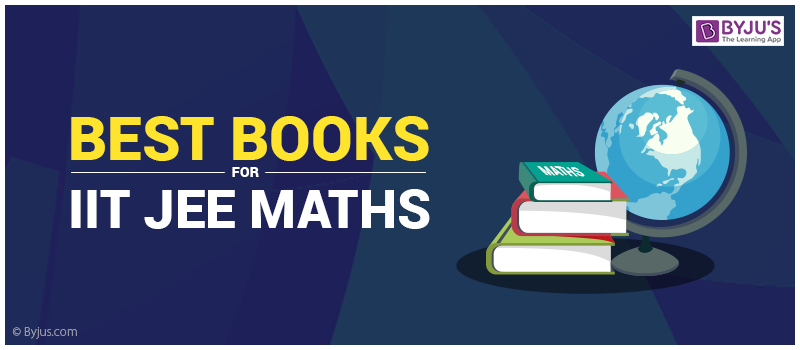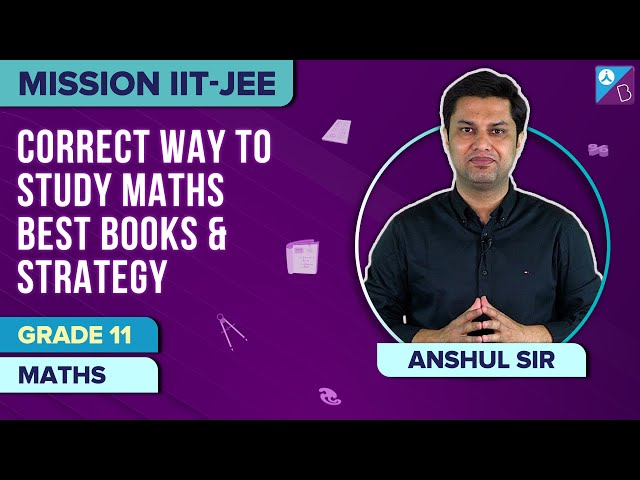# Best Books For IIT JEE Maths (Main and Advanced)Best Books For IIT JEE 2022 – Understanding the concepts and its applications while preparing for JEE Exams is crucial for obtaining better scores, particularly in JEE Main and JEE Advanced. Initially, the prime focus should be on learning each and every concept taught in NCERT textbooks because it is essential to master the basics concepts before moving on to the advanced level. After completing all the exercises in subsequent chapters from NCERT textbooks, you are ready to move to the next stage of IIT JEE preparation.

The next step is to understand the complex and difficult concepts included in JEE Main and JEE Advanced Syllabus that might not be covered in the NCERT textbooks. Candidates can do some research and get some reference books for IIT JEE. This will be helpful especially in learning Maths concepts. Besides, understanding the theoretical portion in Mathematics is equally important as practising different problems, therefore, get a good grip of the theorems and concepts thoroughly before jumping into solving questions. In Maths, you should also focus on understanding how theorems and formulas are derived and the intention behind several conventions and ideas.

## Best Math Books For JEE Main and Advanced

The detailed list of IIT JEE books that can help students in successfully covering the entire JEE Mathematics syllabus is mentioned below:

 Best Book for JEE Maths 1. Course in Mathematics for IIT JEE, Tata McGraw Hill publications 2. Problems Plus in IIT Mathematics, A Das Gupta 3. 36 Years’ Chapterwise Solved Papers, Arihant 4. Class 11th & Class 12th Mathematics, R.D. Sharma 5. IIT Mathematics, M.L. Khanna Unit Best Books Algebra Higher Algebra, Hall and Knight Algebra, Arihant Higher Algebra, Barnard & Child A Textbook of Matrices, Shanti Narayan Trigonometry Trigonometry, S L Loney Calculus Calculus & Analytic Geometry, Thomas & Finney Integral Calculus, Arihant Prakashan Differential Calculus, A Das Gupta Problems in Calculus of One Variable, I. A. Maron Calculus, J. Edward Coordinate Geometry Textbook of Coordinate Geometry, A. Majeed Vector Algebra, Shanti Narayan Co-ordinate geometry, Dr.Gorakh Prasad

It is not mandatory to keep all the above-mentioned books, instead of that, it’s better to analyze each and every topic included in JEE Mathematics syllabus and then make a wise choice of opting for best maths book for JEE Main and Advanced in order to utilize your time efficiently.

## Important and Best Books for IIT JEE Maths### JEE Preparation Tips To Master Mathematics Section

1. After preparing certain topics from NCERT and other advanced textbooks, it’s better to practice several problems from previous year’s question papers for analyzing your understanding of concepts.

2. After solving any problem, always look for the standard solution given in the textbooks. Since most of the concepts included in JEE Syllabus are interlinked, therefore, prepare from the very beginning and don’t skip or start random chapters.

3. Never prepare the same topic from several books. This might lead to the confusion of concepts. Also, do not engage in rote learning or memorizing the concepts. They should learn how to apply the idea to solve a problem.

4. Stay focused and prepare with complete dedication. Candidates can create maths dictionary, flashcards, and even keep a separate notebook for formulas. All these will help them remember important points and concepts easily.

Engaging in rigorous practice is crucial for scoring well in the JEE 2022 Mathematics section. But, the most pressing question is, ‘What should I practice and from Where should I practice’?

BYJU’S – The Learning App has simplified the learning process by consolidating all the best maths book for JEE Main and Advanced in one place. Students can easily learn any particular topic through visualization and practice series of varied problems pertaining to that specific topic anytime and anywhere. Through the visual techniques of problem-solving, students can easily acquire the right skills needed to solve difficult and tricky questions in a minimum time frame.

To access free IIT JEE study materials along with question papers, sample papers and notes, keep visiting BYJU’S.